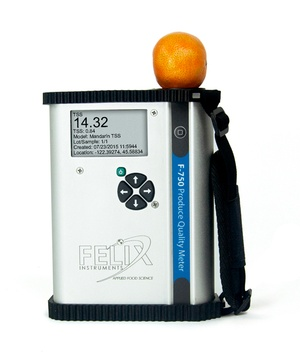# Use Case

### Citrus Application Note

April 20, 2016

Non-destructive measurements of Total Soluble Solids in mandarins

High levels of Total Soluble Solids are positively correlated with consumer preference in citrus, and therefore harvest time should be managed to maximize Total Soluble Solids. Historically, Total Soluble Solids, or “Brix”, have been destructively measured using a refractometer. Near Infrared (NIR) technology, utilized by the F-750 Produce Quality Meter, can assess Total Soluble Solid content non-destructively. To determine the effectiveness and viability of the F-750 Produce Quality Meter in measuring Total Soluble Solids in mandarins, a study was carried out sampling 64 mandarins. Applying the methods adapted from the Felix Instruments Mango Standard Operating Procedure, reference values were correlated with the spectral data collected with the F-750. Results show that the F-750 Produce Quality Meter precisely and non-destructively measures Total Soluble Solids in mandarins, with a calculated root mean square error of cross validation of 0.84 Total Soluble Solids.

Materials and Methods:

In August 2015, 64 mandarins ranging in size and maturity were selected and measured using an F-750 Produce Quality Meter. Next, these same mandarin regions were destructively measured for Total Soluble Solids (TSS) using methods adapted in Felix Instruments Mango Standard Operating Procedure (SOP). These reference values were analyzed alongside the spectral data collected with the instrument using the Model Builder software included with the F-750. A spectral range of 702-975 nm was used with the Model Builder software to detect correlations between the spectral signal and corresponding TSS values. The resulting data were analyzed for linearity, root mean square error, and leave-one-out cross validation error to determine the applicability and accuracy of the created model.Figure 1. F-750 in the process of measuring mandarins.

Results and Discussion:

Results show that the F-750 Produce Quality Meter precisely and non-destructively measures Total Soluble Solids content in mandarins. A strong correlation between spectral data and collected reference values is illustrated by a model prediction R2 of 0.93 (Figure 2). Root Mean Square of Cross Validation (RMSCV) describes the average uncertainty of a model in predicting the total soluble solid content of a new sample. The mandarin model RMSCV value of 0.84 (TSS) demonstrates the accuracy of the model, with an F-750 value of 15 TSS corresponding to an actual total soluble solid value of 14.14-15.86 TSS.Figure 2. Prediction error for mandarins using the F-750. Specimen most strongly correlated to the calculated trend line show the greatest accuracy of prediction (Red: Cross Validation Prediction; Blue: Training Set Prediction

Conclusions:

The F-750 Produce Quality Meter accurately predicted Total Soluble Solids, or “Brix”, in mandarins. Other tests and research findings have demonstrated the viability of using the F-750 to measure other important internal fruit metrics such as total titratable acids and dry matter with the F-750.